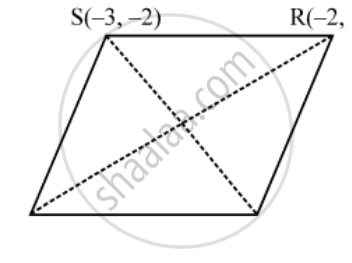Share

# Find the coordinates of the centre of the circle passing through the points (0, 0), (–2, 1) and (–3, 2). Also, find its radius. - CBSE Class 10 - Mathematics

#### Question

If P (2, – 1), Q(3, 4), R(–2, 3) and S(–3, –2) be four points in a plane, show that PQRS is a rhombus but not a square. Find the area of the rhombus

#### Solution

The given points are P(2, –1), Q(3, 4), R(–2, 3) and S(–3, –2).
We have,

PQ=sqrt((3-2)^{2}+(4+1)^{2})=sqrt(1^{2}+5^{2})=\sqrt{26

QR=sqrt((-2-3)^{2}+(3-4)^{2})=\sqrt{25+1}=\sqrt{26}

RS=sqrt((-3+2)^{2}+(-2-3)^{2})=\sqrt{1+25}=\sqrt{26}" units"

SP=sqrt((-3-2)^{2}+(-2+1)^{2})=\sqrt{25+1}=\sqrt{26}" units"

PR=sqrt((-2-2)^{2}+(3+1)^{2})=\sqrt{16+16}=4\sqrt{2}" units"

QS=sqrt((-3-3)^{2}+(-2-4)^{2})=\sqrt{36+36}=6\sqrt{2}" units"

∴ PQ = QR = RS = SP = units and, PR ≠ QSThis means that PQRS is a quadrilateral whose sides are equal but diagonals are not equal.

Thus, PQRS is a rhombus but not a square.

Now, Area of rhombus PQRS = \frac { 1 }{ 2 } × ("Product of lengths of diagonals")

⇒ Area of rhombus PQRS = \frac { 1 }{ 2 } × (PR × QS)

⇒ Area of rhombus PQRS =( \frac{1}{2}\times 4\sqrt{2}\times 6\sqrt{2})sq.units=24sq.units

Is there an error in this question or solution?

#### Video TutorialsVIEW ALL 

Solution Find the coordinates of the centre of the circle passing through the points (0, 0), (–2, 1) and (–3, 2). Also, find its radius. Concept: Distance Formula.
S